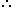Courses

# Test: Calendars- 1

## 10 Questions MCQ Test Logical Reasoning (LR) and Data Interpretation (DI) | Test: Calendars- 1

Description
This mock test of Test: Calendars- 1 for UPSC helps you for every UPSC entrance exam. This contains 10 Multiple Choice Questions for UPSC Test: Calendars- 1 (mcq) to study with solutions a complete question bank. The solved questions answers in this Test: Calendars- 1 quiz give you a good mix of easy questions and tough questions. UPSC students definitely take this Test: Calendars- 1 exercise for a better result in the exam. You can find other Test: Calendars- 1 extra questions, long questions & short questions for UPSC on EduRev as well by searching above.
QUESTION: 1

### The century can end with

Solution:

100 years contain 5 odd days.
∴ Last day of 1st century is Friday.
200 years contain (5 x 2) ≡ 3 odd days.
∴ Last day of 2nd century is Wednesday.
300 years contain (5 x 3) = 15 ≡ 1 odd day.
∴ Last day of 3rd century is Monday.
400 years contain 0 odd day.
∴ Last day of 4th century is Sunday.
This cycle is repeated.
∴ Last day of a century cannot be Tuesday or Thursday or Saturday.

QUESTION: 2

### Find the leap year?

Solution:

Remember the leap year rule
1. Every year divisible by 4 is a leap year, if it is not a century.
2. Every 4th century is a leap year, but no other century is a leap year.
800,1200 and 2000 comes in the category of 4th century (such as 400,800,1200,1600,2000 etc).
Hence 800,1200 and 2000 are leap years

QUESTION: 3

### what was the day of Aug 15 1955?

Solution:
QUESTION: 4

What is the day on July2 1985?

Solution:

Every year has one odd day and a leap year has 2 odd days.
Though 1984 is a leap year, we don't have Feb 29 in the required period. So we get only one odd day and as we are moving back we get Tuesday as the answer.

QUESTION: 5

What was the day of jan 1st 1984?

Solution:

The correct option is Option B.

The first day of 1 January 1984 was Sunday.

QUESTION: 6

Today is Monday. After 61 days, it will be :

Solution:

Each day of the week is repeated after 7 days. So, after 63 days, it will be Monday.
After 61 days, it will be Saturday.

QUESTION: 7

Today is Monday. After 61 days, it will be:

Solution:

Each day of the week is repeated after 7 days.

So, after 63 days, it will be Monday.After 61 days, it will be Saturday.

QUESTION: 8

Q. India got independence on 15th August 1947.what was the day on that date ?

Solution:

We shall first calculate the number of odd days till 31 December 1946.
Number of odd days in the first 1600 years = 0 odd day
Number of odd days in the next 300 years = 1 odd day
Now, 46 years had 11 leap years and 35 ordinary years.
The number of odd days in 46 years=(2×11)+(1×35)=22+35=57=8weeks and 1 odd day.
Now, we shall calculate the number of odd days in 1947 till 15 August.
Month Days January(31) February(28) March(31) April(30) May(31) June(30) July(31) August(15)
Days = 31 + 28 + 31 + 30 + 31 + 30 + 31 + 15 = 227
227 days = 32 weeks and 3 odd days
So, the total number of odd days till 15 August 1947 = 0 + 1 + 1 + 3 = 5
On counting five days from Monday, we get Friday.
Therefore, 15 August 1947 was a Friday.

QUESTION: 9

Which calendar year will be same as the year 2008?

Solution:

For every 28 years the calendars will same, so years 2008,2036 have same calendar like 1980

QUESTION: 10

The day of the 5th november is equal to the day of the date in the same year?

Solution:

We will show that the number of odd days between the last day of February and the last day of October is zero.
March April May June July Aug. Sept. Oct.
31 + 30 + 31 + 30 + 31 + 31 + 30 + 31 = 241 days = 35 weeks = 0 odd day.
Number of odd days during this period = 0.
Thus, 5th March of a year will be the same day as 5th November of that year.